International
Tables for
Crystallography
Volume A
Space-group symmetry
Edited by M. I. Aroyo

International Tables for Crystallography (2016). Vol. A, ch. 3.2, pp. 720-776
https://doi.org/10.1107/97809553602060000930

Chapter 3.2. Point groups and crystal classes

Th. Hahn,a H. Klapper,a* U. Müllerb* and M. I. Aroyoc

aInstitut für Kristallographie, RWTH Aachen University, 52062 Aachen, Germany,bFachbereich Chemie, Philipps-Universität, D-35032 Marburg, Germany, and cDepartamento de Física de la Materia Condensada, Universidad del País Vasco (UPV/EHU), Bilbao, Spain
Correspondence e-mail:  klapper@ifk.rwth-aachen.de, mueller@chemie.uni-marburg.de

Footnotes

Deceased.

1 For reasons of simplicity, in this chapter the same term point group' is used for a particular point group' and a type of point group'. For space groups, this distinction is explained in Section 1.3.4.1. For a different use of the term point group' see Section 1.3.3.1.
2 This does not apply to rhombohedral axes': here the positive directions of all three axes slope upwards from the plane of the paper: cf. Fig. 2.1.3.9.
3 Dual polyhedra have the same number of edges, but the numbers of faces and vertices are interchanged; cf. textbooks of geometry.
4 For a survey of these relations, as well as of the limiting forms', it is helpful to consider the (seven) normalizers of the crystallographic point groups in the group of all rotations and reflections (orthogonal group, sphere group); normalizers of the crystallographic and noncrystallographic point groups are listed in Tables 3.5.4.1and 3.5.4.2.
5 The treatment of limiting forms' in the literature is quite ambiguous. In some textbooks, limiting forms are omitted or treated as special forms in their own right: other authors define only limiting general forms and consider limiting special forms as if they were new special forms. For additional reading, see P. Niggli (1941, pp. 80–98).
6 The topology of a polyhedron is determined by the numbers of its vertices, edges and faces, by the number of vertices of each face and by the number of faces meeting in each vertex.
7 For the exceptions in the cubic crystal system cf. Section 3.2.1.2.4, Notes on crystal and point forms, item (viii).
8 The matrices of corresponding tripletsand, i.e. of triplets generated by the same symmetry operation from (hkl) and x, y, z, are inverse to each other, provided the x, y, z andare regarded as columns and the (hkl) andas rows: this is due to the contravariant and covariant nature of the point coordinates and Miller indices, respectively. Note that for orthogonal matrices the inverse matrix equals the transposed matrix; in crystallography, this applies to all coordinate systems (including the rhombohedral one), except for the hexagonal system. The matrices for the symmetry operations occurring in the crystallographic point groups are listed in Tables 1.2.2.1and 1.2.2.2.
9 Thus, the name prism' for a point form implies combination of the prism with a pinacoid.
10 A tetragonal tetrahedron is a digonal streptohedron; hence, its dual is a digonal antiprism', which is again a tetragonal tetrahedron.
11 The dual of a tetragonal (= di-digonal) scalenohedron is a digonal antiprism', which is cut off' by the pinacoid.
12 This type of diagram was first used in International Tables for the Determination of Crystal Structures (1935): in International Tables for X-ray Crystallography (1952)a somewhat different approach was employed.
13 The classes of general point groups' are not the same as the commonly used crystal classes' because some of them contain point groups of different orders. All these orders, however, follow a common scheme. In this sense, the general classes' are an extension of the concept of (geometric) crystal classes. For example, the general class nmm of the 4N-gonal system contains the point groups 4mm (tetragonal), 8mm (octagonal), 12mm (dodecagonal), 16mm etc.
14 The axes of order infinity, as considered here, do not correspond to cyclic groups (as do the axes of finite order) because there is no smallest rotation from which all other rotations can be derived as higher powers, i.e. by successive application. Instead, rotations of all possible angles exist. Nevertheless, it is customary to symbolize these axes as ∞ or; note that the Hermann–Mauguin symbolsandare equivalent, and so are the Schoenflies symbolsand. (There exist also axes of order infinity that do correspond to cyclic groups, namely axes based upon smallest rotations with irrational values of the rotation angle.)
15 Here, the-gonal and the-gonal systems are distinguished in order to bring out the analogy with the trigonal and the hexagonal crystal systems. They could equally well be combined into one, in correspondence with the hexagonal crystal family' (cf. Sections 1.3.4.4and 2.1.1).
16 The terms rotating' and stationary' in the circular, cylindrical and spherical systems do not imply any relation to dynamical properties (motions) of crystals or molecules. They only serve to illustrate the absence (group) or presence (,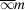) of vertical' mirror planes in these groups or order.
17 The noncrystallographic face and point forms are extensions of the corresponding crystallographic forms: cf. Section 3.2.1.2.4, Notes on crystal and point forms. The name streptohedron applies to the general face forms of point groupswithand; it is thus a generalization of the tetragonal disphenoid or tetragonal tetrahedronand the rhombohedron.
18 The term Miller indices' is used here also for the noncrystallographic point groups. Note that these indices do not have to be integers or rational numbers, as for the crystallographic point groups. Irrational indices, however, can always be closely approximated by integers, quite often even by small integers.
19 A one-step procedure applies to the icosahedral Wyckoff position' 12a, the face poles and points of which are located on the fivefold axes. Here, step (ii) is redundant and can be omitted. The formsandare contained in the cubic point groups 23 andand in the cubic space groups P23 andas limiting cases of Wyckoff positionsand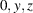with specialized (irrational) values of the indices and coordinates. In geometric terms, the regular pentagon-dodecahedron is a noncrystallographic limiting polyhedron' of the crystallographic' pentagon-dodecahedron and the regular icosahedron is a limiting polyhedron' of the irregular' icosahedron (cf. Section 3.2.1.2.2, Crystal and point forms).
20 Note that for orthogonal matrices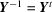(t = transposed).
21 The number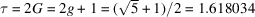(Fibonacci number) is the characteristic value of the golden section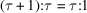, i.e.. Furthermore, τ is the distance between alternating vertices of a regular pentagon of unit edge length and the distance from centre to vertex of a regular decagon of unit edge length.

22 It should be noted, however, that asymmetric etch figures may occur that are due, for example, to an inclination of dislocation lines against the surface.
23 This property can be represented by enantiomorphic spheres of point group 2, cf. Table 3.2.1.6.
24 In the literature, the requirement for pyroelectricity is frequently expressed as a unique (or singular) polar axis'. This term, however, is misleading for point groups 1 and m.

25 In the IUPAC Recommendations 2011, a different notation for polymeric helices is recommended, in that a 7/2 helix be named 72 helix (Meille et al., 2011). This is very unfortunate because it causes confusion with the Hermann–Mauguin symbols: the Hermann–Mauguin symbol of the 7/2 helix is not 72, but 74 or 73.
26 Many structural researchers are not aware of the difference between chiral (enantiomorphic) space groups and Sohncke space groups; frequently, even in textbooks, the term chiral space group' is used although an achiral Sohncke space group is meant.